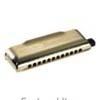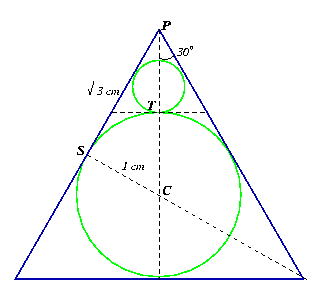#### You may also like### Generally Geometric

Generalise the sum of a GP by using derivatives to make the coefficients into powers of the natural numbers.### Harmonically

Is it true that a large integer m can be taken such that: 1 + 1/2 + 1/3 + ... +1/m > 100 ?

##### Age 16 to 18Challenge Level

Thank you Tom from Wolgarston High School, Hidayah who does not name a school, Hamish and Jared from Ulverston Victoria High School, Eduardo from The British School, Manila, Justin from Skyview High School, Billings, MT USA and Fabian from Sabino High School in Tucson, Arizona, U.S.A. for sending your very good solutions to this problem.You all tackled the problem in a similar way.Let $C$ be the centre of the central circle. Then $PS$ is a tangent to this circle. Then triangle $CSP$ is a 30-60-90 triangle and, given that $CS = 1$ cm, it follows that $PS = \sqrt{3}$ cm and $PC = 2$ cm. Hence the height of the largest equilateral triangle is $3$ cm. ($PC$ + radius of $1$ cm.), the height of the next size equilateral triangle is $1$ cm. ($PC$ - radius of $1$ cm.) and the radius of the next size circle is $1/3$ cm.

Each circle is scaled down by a linear scale factor of $1/3$ and by an area scale factor of $1/9$.

There is one central circle and at each stage after that 3 new circles are added.

The 'total circumference' is the sum of the circumferences of all the circles, given by:

Total circumference = $2\pi + 3 ({ 2\pi\over 3} + {2\pi\over 9} + {2\pi \over 27}+ \ldots )$

where the dots denote that the sum goes on for ever. Summing this infinite geometric series gives: $$\mbox{Total circumference} = 2\pi + 2\pi (1 + {1\over 3} + {1\over 9} + {1\over 27} + \ldots ) = 2\pi + \frac{2\pi}{1 - 1/3} = 5\pi$$ The 'total area' is the sum of the areas of all the circles, given by:

Total area = $\pi + 3 ({\pi\over 9} + {\pi\over 9^2} + {\pi\over 9^3} + \ldots )$

where the dots denote that the sum goes on for ever. Summing this infinite geometric series gives: $$\mbox{Total area} = \pi + {\pi\over 3} (1 + {1\over 9} + {1\over 9^2} + \ldots ) = \pi + \frac{\pi}{3(1 - 1/9)} = \frac{11\pi}{8}$$ Clearly, the total of the circumferences grows faster than the total of the areas because the scale factor 1/3 is bigger than the scale factor 1/9.